Скачать презентацию Some Financial Mathematics The one-period rate of

d3b8e7bbb1441b402de64cde8bd00a34.ppt

• Количество слайдов: 20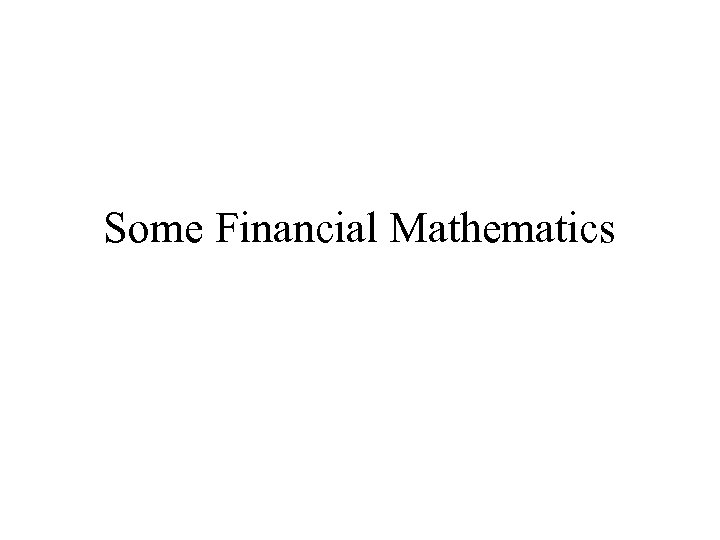Some Financial Mathematics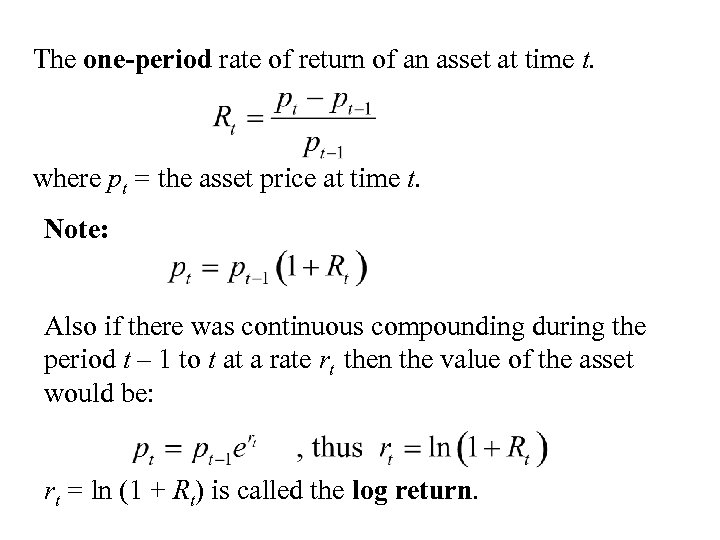The one-period rate of return of an asset at time t. where pt = the asset price at time t. Note: Also if there was continuous compounding during the period t – 1 to t at a rate rt then the value of the asset would be: rt = ln (1 + Rt) is called the log return.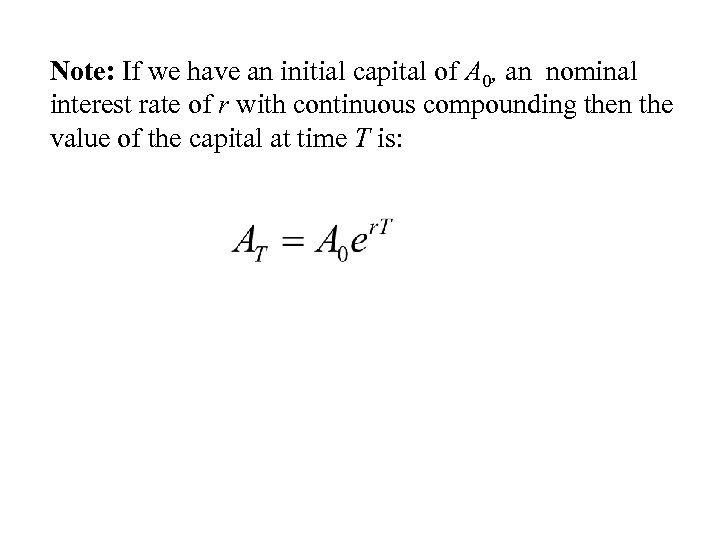Note: If we have an initial capital of A 0, an nominal interest rate of r with continuous compounding then the value of the capital at time T is: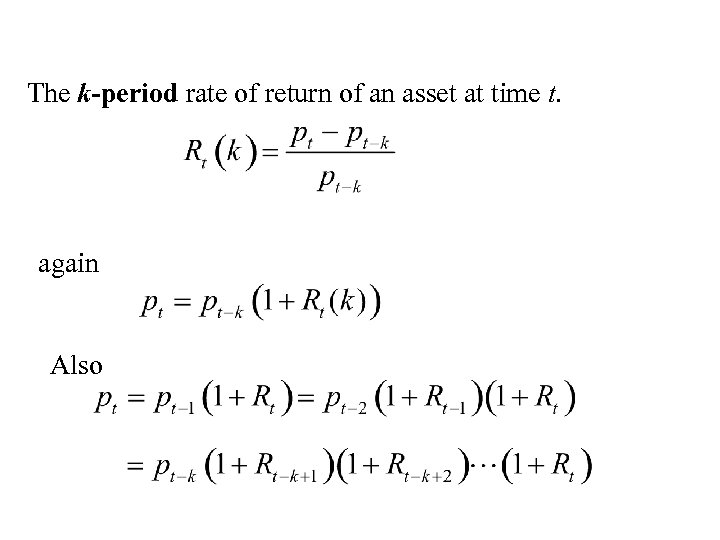The k-period rate of return of an asset at time t. again Also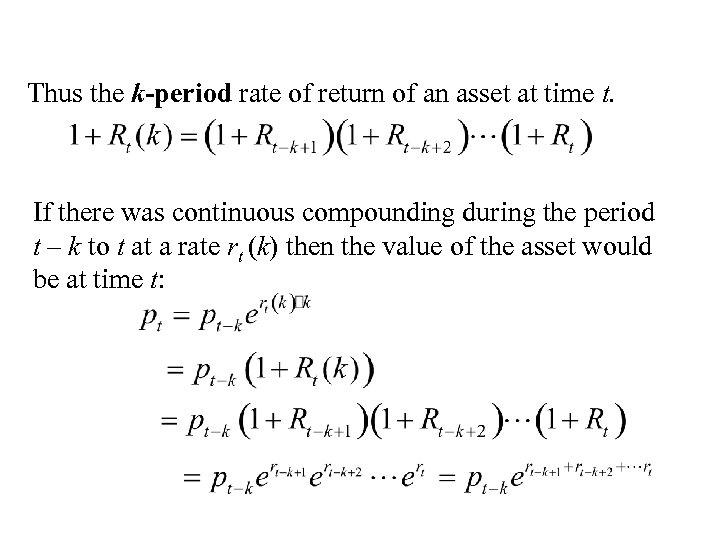Thus the k-period rate of return of an asset at time t. If there was continuous compounding during the period t – k to t at a rate rt (k) then the value of the asset would be at time t: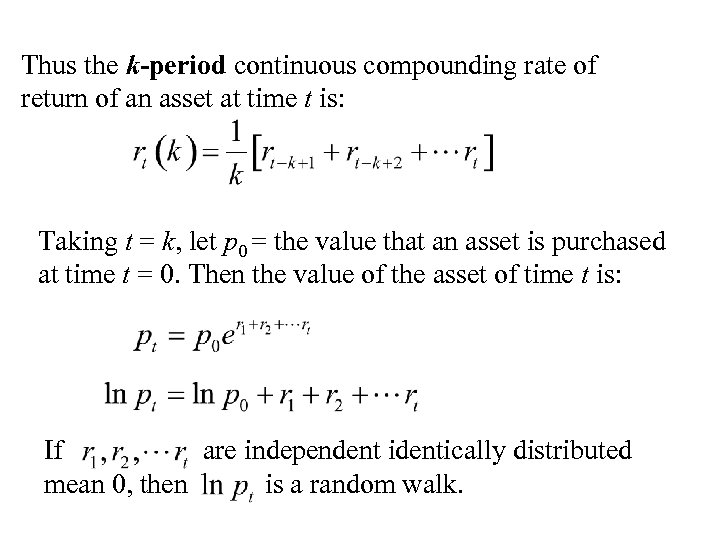Thus the k-period continuous compounding rate of return of an asset at time t is: Taking t = k, let p 0 = the value that an asset is purchased at time t = 0. Then the value of the asset of time t is: If are independent identically distributed mean 0, then is a random walk.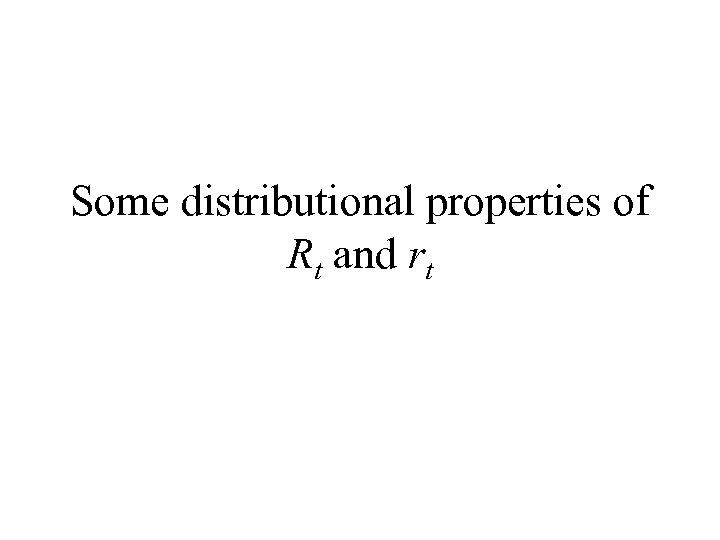Some distributional properties of Rt and rt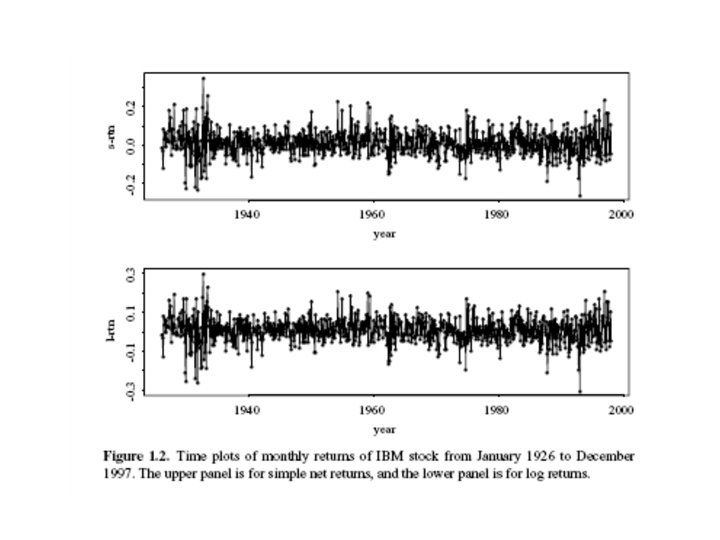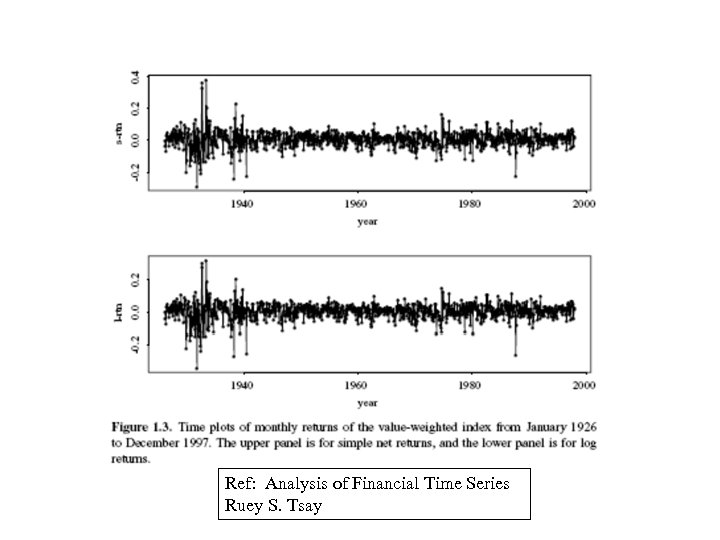Ref: Analysis of Financial Time Series Ruey S. Tsay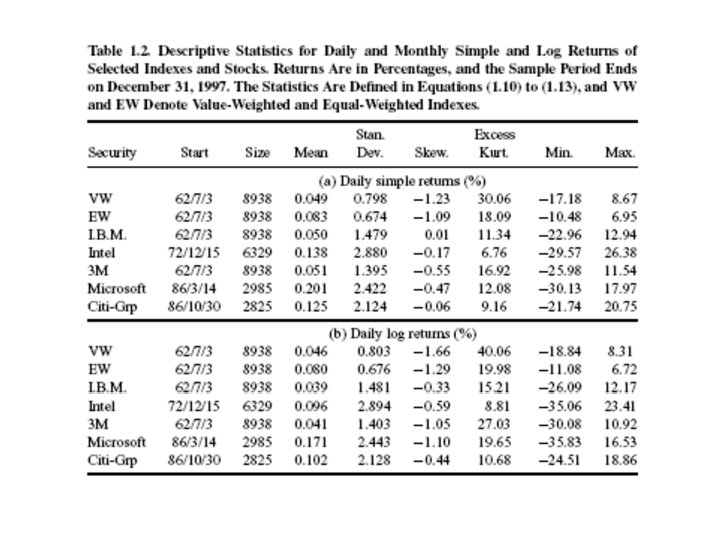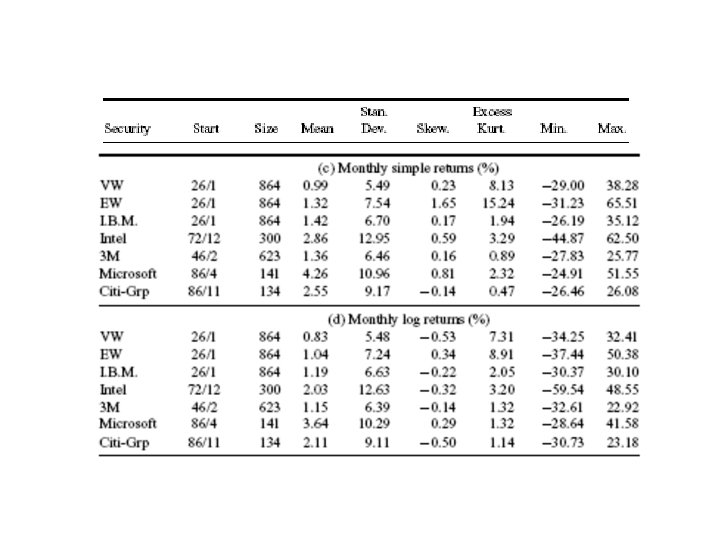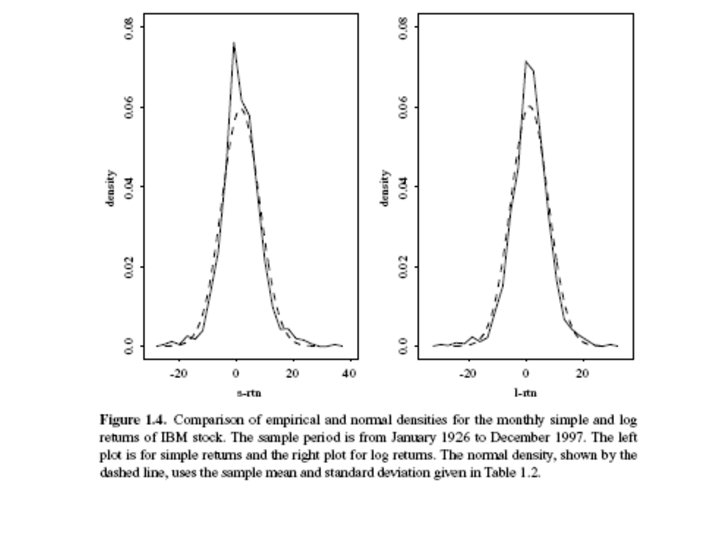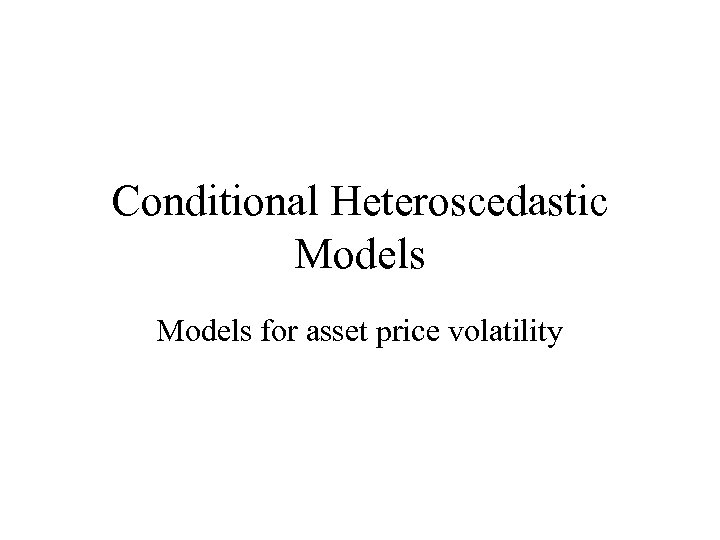Conditional Heteroscedastic Models for asset price volatility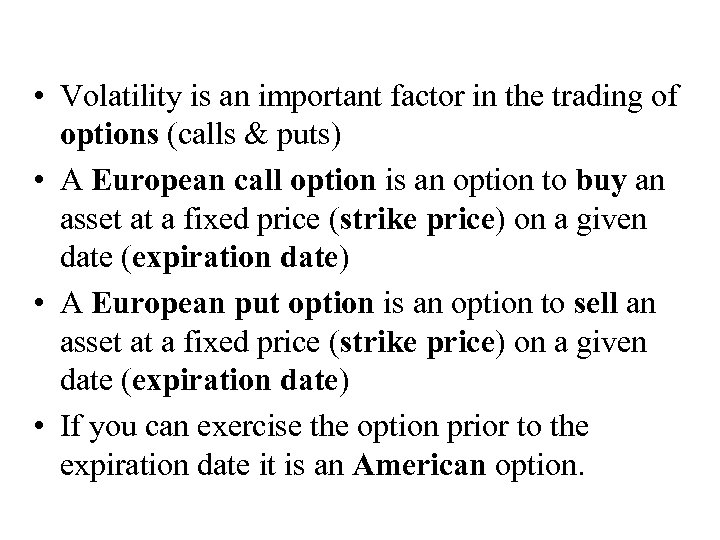• Volatility is an important factor in the trading of options (calls & puts) • A European call option is an option to buy an asset at a fixed price (strike price) on a given date (expiration date) • A European put option is an option to sell an asset at a fixed price (strike price) on a given date (expiration date) • If you can exercise the option prior to the expiration date it is an American option.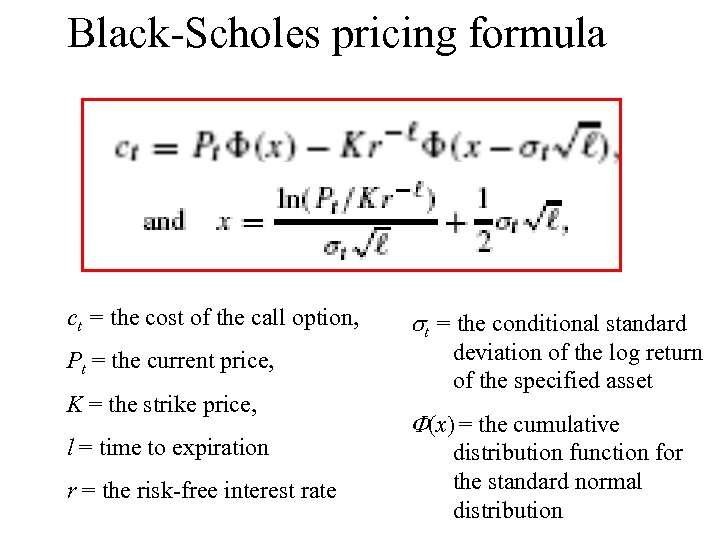Black-Scholes pricing formula ct = the cost of the call option, Pt = the current price, K = the strike price, l = time to expiration r = the risk-free interest rate st = the conditional standard deviation of the log return of the specified asset F(x) = the cumulative distribution function for the standard normal distribution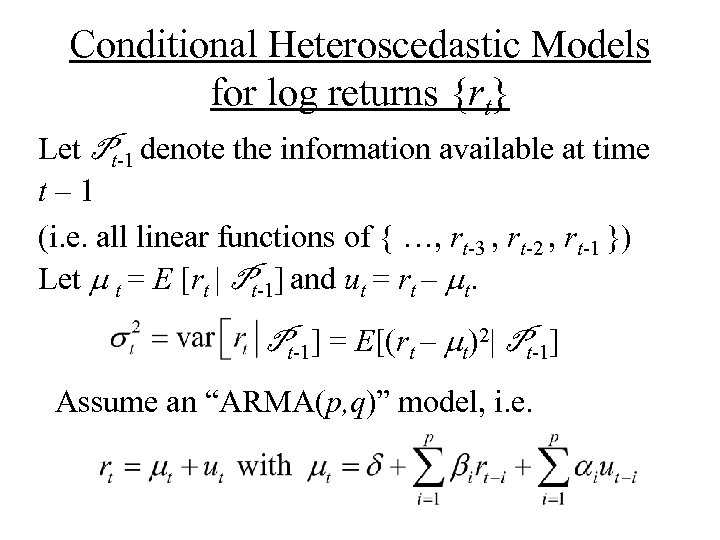Conditional Heteroscedastic Models for log returns {rt} Let Pt-1 denote the information available at time t– 1 (i. e. all linear functions of { …, rt-3 , rt-2 , rt-1 }) Let m t = E [rt | Pt-1] and ut = rt – mt. Pt-1] = E[(rt – mt)2| Pt-1] Assume an “ARMA(p, q)” model, i. e.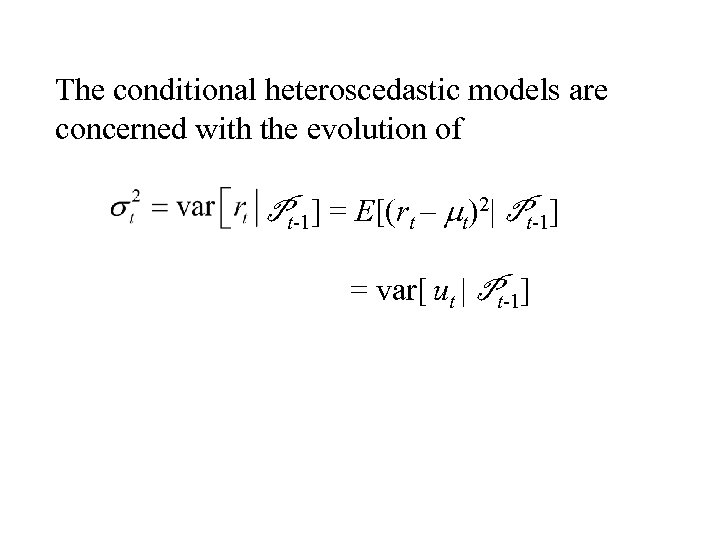The conditional heteroscedastic models are concerned with the evolution of Pt-1] = E[(rt – mt)2| Pt-1] = var[ ut | Pt-1]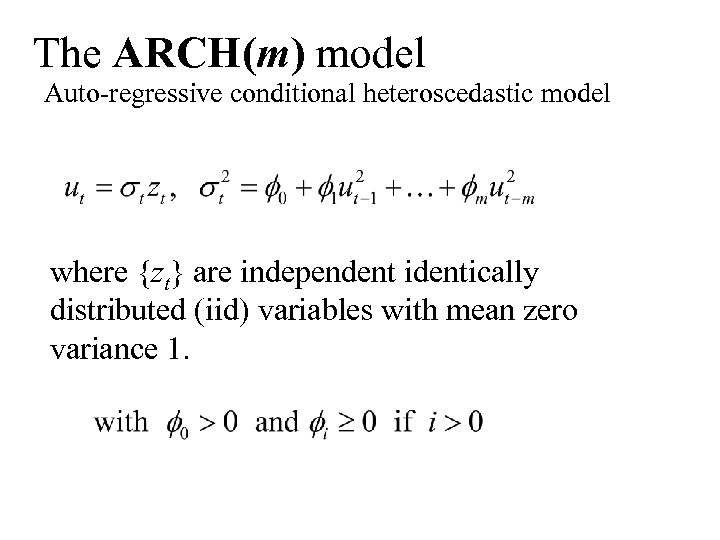The ARCH(m) model Auto-regressive conditional heteroscedastic model where {zt} are independent identically distributed (iid) variables with mean zero variance 1.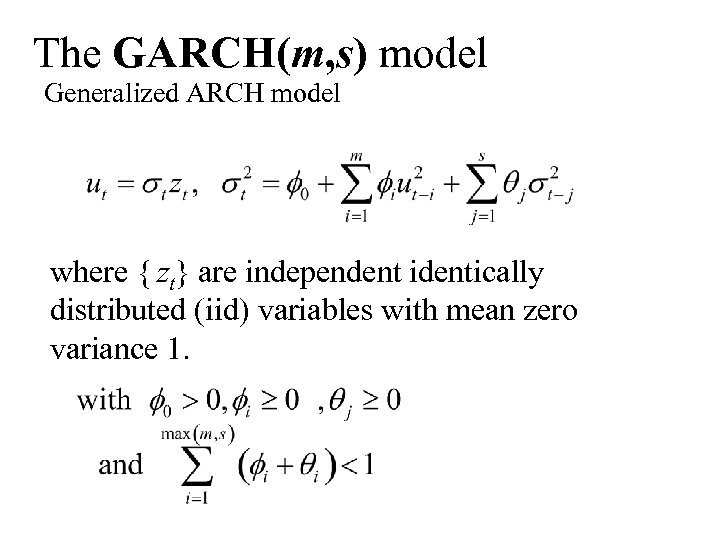The GARCH(m, s) model Generalized ARCH model where { zt} are independent identically distributed (iid) variables with mean zero variance 1.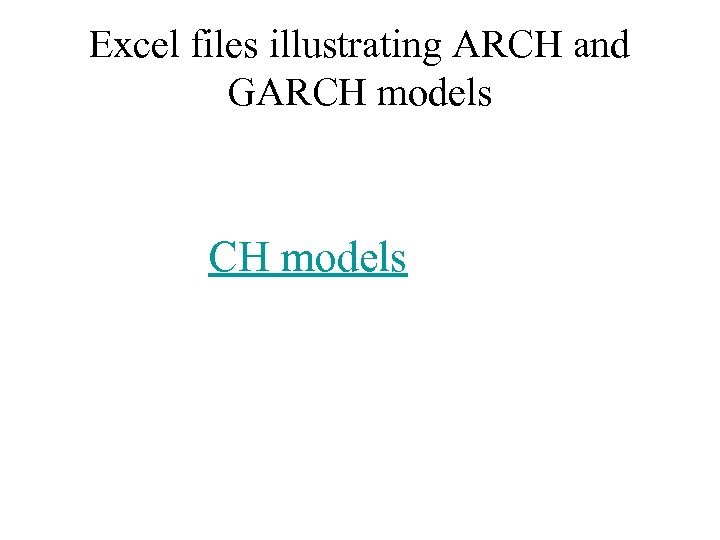Excel files illustrating ARCH and GARCH models